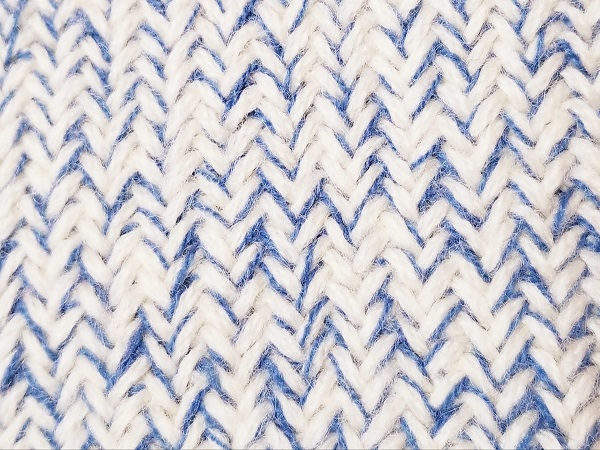KNIT MAGAZINE

# How do you know the knit mix ratio? ｜ Calculation method of total mixing ratioHello, this is Akaishi from Maruyasu Yarn.

This time,

Mixing ratio of clothes = what material is used and how much

How do you calculate it for knits?

I would like to explain about.

## The knit mixing ratio is expressed as the total mixing ratio.

The quality label on your clothes shows the mixing ratio.

50% cotton

50% polyester

It’s like that.

However, if you align the threads or mix various threads with a JQ pattern etc.,

A material 50% cotton 50% polyester

B material 100% polyester

Cannot be written.

In knitting, when we knit together using several types of threads, we consider it as one mixed material, calculate the ratio of each material (mixing ratio), and calculate the “total mixing ratio”.

* Of course, if the materials are separated by parts such as sleeves and collar, the notation for each part is OK.

## How to calculate the total mixing ratio

The following data is required for the calculation of the total mixing ratio.

① Weight of the whole product (Metsuke)

② Weight of each thread (Metsuke)

③ Mixing ratio of each thread

As an example, consider the following.

① Weight of the whole product 300g

A material: 150g

B material: 150g

③ Mixing ratio of each thread

A material: 50% cotton 50% polyester

B material: 100% hair

From the weight of each thread, determine how many grams of material the thread is.

From the amount of thread in ②, calculate how much each material is used.

A material: 150g x 50% cotton = 75g (150 x 0.5)

150g x 50% polyester = 75g (150 x 0.5)

B material: 150g x 100% hair = 150g

In summary, each material in this product is

Cotton 75g

Polyester 75g

Hair 150g

It turns out that the above amount is used.

Calculate what percentage of each material is in the overall product

Next, calculate the percentage of material.

Weight of each material ÷ Weight of the entire product in ①

75g ÷ 300g = 25% cotton

Polyester 75g ÷ 300g = Polyester 25%

Hair 150g ÷ 300g = 50%

With this, the total mixing ratio has come out.

The total mixing ratio is written in order from the one with the highest mixing ratio, so it is as follows.

Hair 50%

Cotton 25%

25% polyester

## summary

What did you think?

It’s easy once you understand how to do it, but if you haven’t used it for a while, you may forget it.

Also, when deciding the notation, it is easy to carelessly when plating the stretch yarns Dusley or Marlon.

There is no problem if it is only a small amount such as ribs or knitting, but it is not necessary to indicate it on the entire surface, but since the amount of stretch yarn that needs to be indicated is used on the entire surface, let’s put it out with the total mixing ratio.